# Homework 8 Functional Visitors in DJ Use functional

• Slides: 13Homework 8 Functional Visitors in DJ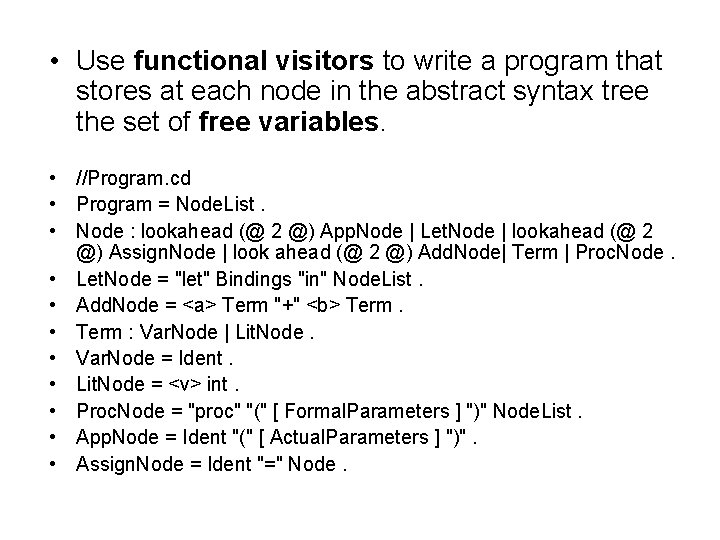• Use functional visitors to write a program that stores at each node in the abstract syntax tree the set of free variables. • //Program. cd • Program = Node. List. • Node : lookahead (@ 2 @) App. Node | Let. Node | lookahead (@ 2 @) Assign. Node | look ahead (@ 2 @) Add. Node| Term | Proc. Node. • Let. Node = "let" Bindings "in" Node. List. • Add. Node = <a> Term "+" <b> Term. • Term : Var. Node | Lit. Node. • Var. Node = Ident. • Lit. Node = <v> int. • Proc. Node = "proc" "(" [ Formal. Parameters ] ")" Node. List. • App. Node = Ident "(" [ Actual. Parameters ] ")". • Assign. Node = Ident "=" Node.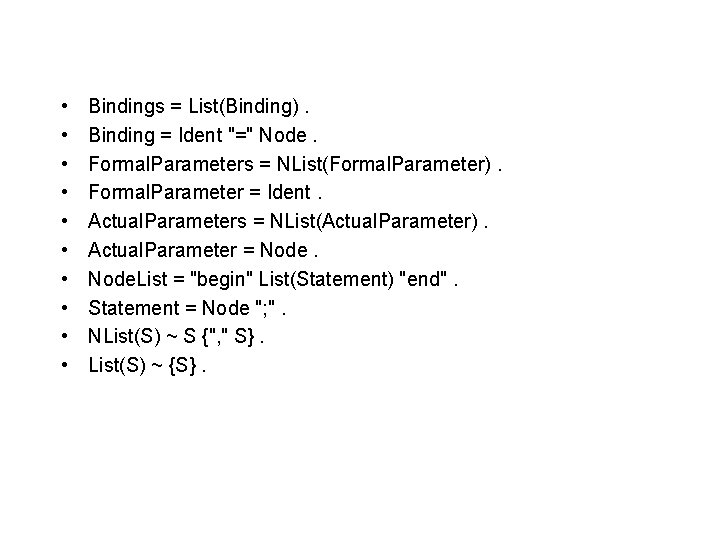• • • Bindings = List(Binding). Binding = Ident "=" Node. Formal. Parameters = NList(Formal. Parameter). Formal. Parameter = Ident. Actual. Parameters = NList(Actual. Parameter). Actual. Parameter = Node. List = "begin" List(Statement) "end". Statement = Node "; ". NList(S) ~ S {", " S}. List(S) ~ {S}.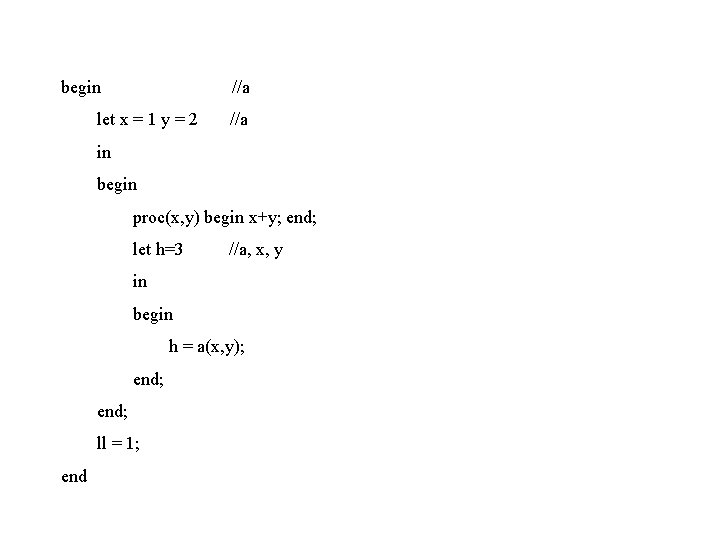begin //a let x = 1 y = 2 //a in begin proc(x, y) begin x+y; end; let h=3 //a, x, y in begin h = a(x, y); end; ll = 1; endFunctional Visitors • Enable functional-style computation combinations, which are desirable for a lot of recursive computation scenarios. • Object combine(Object[] values)specify the desired computation combination. • Enable the flexibility of users to control the traversal according to the computation of Visitor itself. • Object apply(String label) go down to the edge labeled as label and return the value from the sub traversal;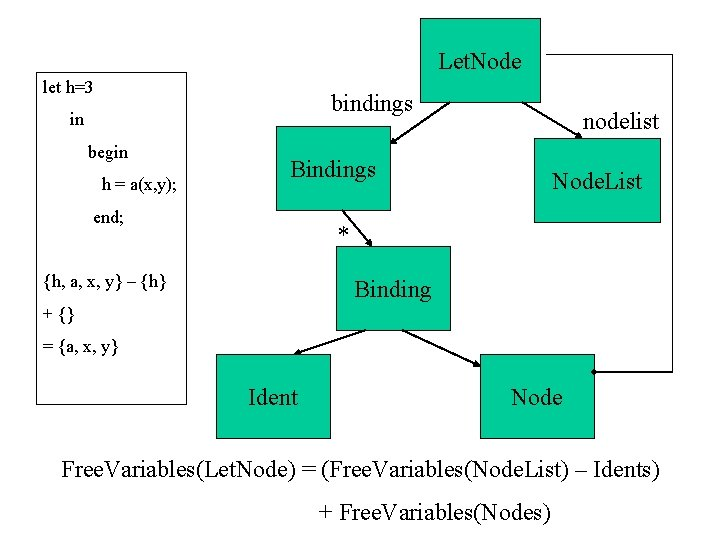Let. Node let h=3 bindings in begin h = a(x, y); Bindings end; nodelist Node. List * {h, a, x, y} – {h} Binding + {} = {a, x, y} Ident Node Free. Variables(Let. Node) = (Free. Variables(Node. List) – Idents) + Free. Variables(Nodes)Solution one /proj/demeter/com 3362/w 02/students/wupc] 1: dehg fine around method to class Let. Node, Ident, Lit. Node, Binding, Proc. Node, Formal. Parameter. 2. Use List to store free variables, and Hash. Map to store bindingsSolution two /proj/demeter/com 3362/w 02/students/lbenitez 1. Use a stack to store the nodes visited in the traversal, and a stack to store the bindings. 2. Define around method to class Node. List to compute free variables of the nodes in the node stack. 3. Define before and after methods to class Let. Node, Proc. Node to add a new binding field to the binding stack 4. Define before method to class Binding and Formal. Parameter to add a new binding to the current binding field 5. Define before method to subclasses of class Node to store the free variables of this node into the node stack.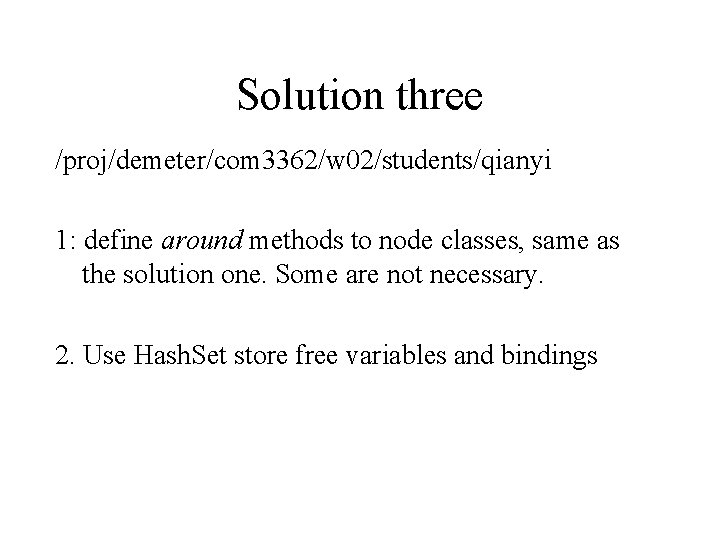Solution three /proj/demeter/com 3362/w 02/students/qianyi 1: define around methods to node classes, same as the solution one. Some are not necessary. 2. Use Hash. Set store free variables and bindings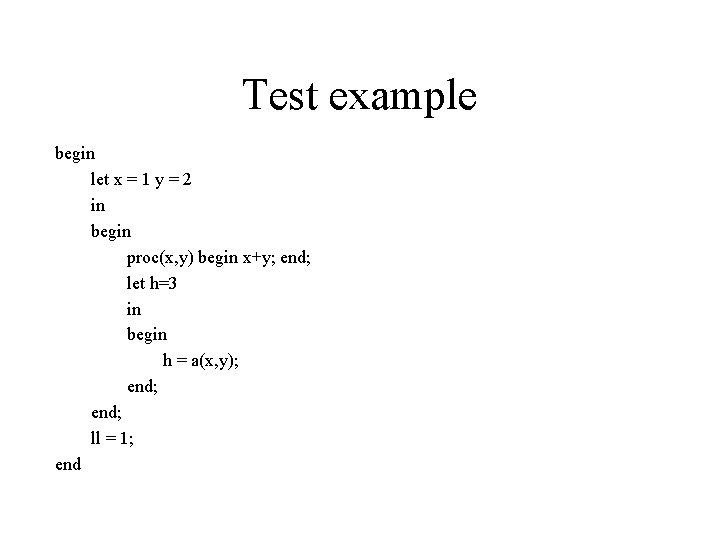Test example begin let x = 1 y = 2 in begin proc(x, y) begin x+y; end; let h=3 in begin h = a(x, y); end; ll = 1; end• • • • • Lit. [email protected] d 49() Lit. [email protected] a 3() Var. [email protected] d 386(x) Var. [email protected] f 1 d(y) Add. [email protected] e 059(x, y, ) Node. [email protected](x, y, ) Proc. [email protected] c 3(x, y, ) Lit. [email protected] c 0() Var. [email protected](x) Var. [email protected] f 6 cd(y) App. [email protected] bb 514(x, a, y, ) Assign. [email protected] d 5 d 2 a(x, a, y, ) Node. [email protected] fa 474(x, a, y, ) Let. [email protected] c 083(x, a, y, ) //2 Node. [email protected] d 8 c 1(x, a, y, ) Let. [email protected] d 9 c 06(a, ) //1 Lit. [email protected] b 6889() Assign. [email protected] 2 ff 5() Node. [email protected] e(a, )Caching aspect • • Parent Chind Caching Back My. Caching My. Back Free. Variable. CachingCaching aspect • pointcut caching(Parent c): call(void universal_trv 0(. . )) && target(c); //? ? • Question: When we use a functional visitor in a traversal, which method is called of every node visited by this visitor.